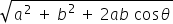science
Easy

Question

# If two vectors moved 5 m and 5 m, respectively, to the east and north direction, and the angle between the vectors is 90 degrees, then find the direction of the resultant?

## The correct answer is: 45

science

science

### Two vectors started from the origin and moved to the east and north direction 10 m, 15 m, respectively, and the angle between the vectors is 30 degrees, then find the resultant of the vectors.

If two vectors A and B with magnitude a and b respectively are given and the angle between them is θ Then the magnitude of the Resultant of A and B is R =### Two vectors started from the origin and moved to the east and north direction 10 m, 15 m, respectively, and the angle between the vectors is 30 degrees, then find the resultant of the vectors.

If two vectors A and B with magnitude a and b respectively are given and the angle between them is θ Then the magnitude of the Resultant of A and B is R =science

### If a car moves 10 m in the north direction and 5 m in the west direction, then find the resultant.science

science

science

### In the parallelogram law of vectors, the cos angle denotedscience

science

science

### Which of the following is a vector quantity?science

science

science

### Find the resultant of the vector if two orthogonal vectors are of magnitude 10 m and 20 m.science

### If the vectors are of the same magnitude and same direction, they are said to be

Two vectors are said to be equal if they have same magnitude and direction, regardless of the positions of their positions of their initial points.

### If the vectors are of the same magnitude and same direction, they are said to be

Two vectors are said to be equal if they have same magnitude and direction, regardless of the positions of their positions of their initial points.

science

### The angle between the vectors is _______ if they are orthogonal

Two vectors are orthogonal when they are perpendicular to each other. i.e. 90 degrees and the dot product of the two vectors is 0.

### The angle between the vectors is _______ if they are orthogonal

Two vectors are orthogonal when they are perpendicular to each other. i.e. 90 degrees and the dot product of the two vectors is 0.

science

### The triangle law of vectors is used for#### With Turito Foundation.#### Get an Expert Advice From Turito.